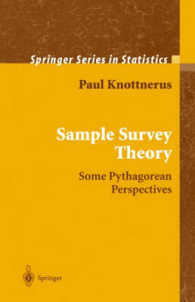### Sample Survey Theory : Some Pythagorean Physics (Springer Series in Statistics) （2002. 415 p.）

• 提携先の海外書籍取次会社に在庫がございます。通常3週間で発送いたします。
重要ご説明事項
1. 納期遅延や、ご入手不能となる場合が若干ございます。
2. 複数冊ご注文の場合、分割発送となる場合がございます。
3. 美品のご指定は承りかねます。
• 【重要：入荷遅延について】
ウクライナ情勢悪化・新型コロナウィルス感染拡大により、洋書・洋古書の入荷が不安定になっています。詳しくはこちらをご確認ください。
海外からのお取り寄せの場合、弊社サイト内で表示している標準的な納期よりもお届けまでに日数がかかる見込みでございます。
申し訳ございませんが、あらかじめご了承くださいますようお願い申し上げます。
• ◆画像の表紙や帯等は実物とは異なる場合があります。
• 製本 Hardcover:ハードカバー版／ページ数 415 p.
• 商品コード 9780387954073

### Full Description

This book describes a novel approach to the theory of sampling from finite populations. The new unifying approach is based on the sampling autocorrelation coefficient. The author derives a general set of sampling equations that describe the estimators, their variances as well as the corresponding variance estimators. This volume will be useful for survey practitioners faced with complex surveys.

### Contents

Introduction and Outline of the Book * Elementary Statistics * Alternative Approach to Unequal Probability Sampling * A General Rho Theory on Survey Sampling * Variance Estimation for Some Standard Designs * Multistage and Cluster (Sub)Sampling * Systematic Sampling * Estimation of the Sampling Autocorrelation Coefficient * Variance Approximations * A Simulation Study * The Regression Estimator Revisited * General Restriction Estimator in Multisurvey Sampling * Weighting Procedures